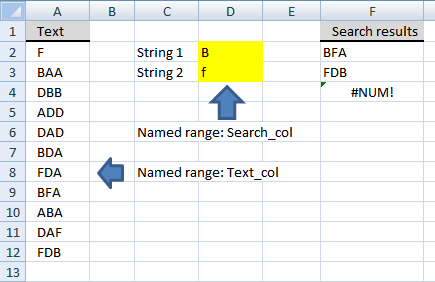Author: Oscar Cronquist Article last updated on December 02, 2018

Question: How do I search a list for two text strings and return a list with where both strings occurs?

Answer: Here is a formula (F2:F4) that creates a list with text values where both strings occur and is not case-sensitive.### Array formula in cell F2

=INDEX(Text_col, SMALL(IF(FREQUENCY(IF(ISERR(SEARCH(TRANSPOSE(Search_col), Text_col)), "", ROW(Text_col)-MIN(ROW(Text_col))+1), ROW(Text_col)-MIN(ROW(Text_col))+1)=ROWS(Search_col), ROW(Text_col)-MIN(ROW(Text_col))+1, ""), ROW(1:1))) + CTRL + SHIFT + ENTER.

Copy cell F2 and paste down as far as needed.

### Alternative array formula in cell F2

=INDEX(Text_col, SMALL(IF(ISERR(SEARCH(\$D\$2, Text_col)*SEARCH(\$D\$3, Text_col)), "", ROW(Text_col)-MIN(ROW(Text_col))+1), ROW(1:1))) + CTRL + SHIFT + ENTER.

Copy cell F2 and paste down as far as needed.

### Explaining array formula in cell F2

=INDEX(Text_col, SMALL(IF(FREQUENCY(IF(ISERR(SEARCH(TRANSPOSE(Search_col), Text_col)), "", ROW(Text_col)-MIN(ROW(Text_col))+1), ROW(Text_col)-MIN(ROW(Text_col))+1)=ROWS(Search_col), ROW(Text_col)-MIN(ROW(Text_col))+1, ""), ROW(1:1)))

Step 1 - Find cells containing both strings

=INDEX(Text_col, SMALL(IF(FREQUENCY(IF(ISERR(SEARCH(TRANSPOSE(Search_col), Text_col)), "", ROW(Text_col)-MIN(ROW(Text_col))+1), ROW(Text_col)-MIN(ROW(Text_col))+1)=ROWS(Search_col), ROW(Text_col)-MIN(ROW(Text_col))+1, ""), ROW(1:1)))

SEARCH(find_text, within_text, [start_num]) returns the number of the character at which a specific character or text string is first found, reading left to right (not case sensitive)

SEARCH(TRANSPOSE(Search_col), Text_col))

becomes

becomes

becomes

{#VALUE!, 1;1, #VALUE!;2, #VALUE!;#VALUE!, #VALUE!;#VALUE!, #VALUE!;1, #VALUE!;#VALUE!, 1;1, 2;2, #VALUE!;#VALUE!, 3;3, 1}

Step 2 - Convert numbers to row numbers

=INDEX(Text_col, SMALL(IF(FREQUENCY(IF(ISERR(SEARCH(TRANSPOSE(Search_col), Text_col)), "", ROW(Text_col)-MIN(ROW(Text_col))+1), ROW(Text_col)-MIN(ROW(Text_col))+1)=ROWS(Search_col), ROW(Text_col)-MIN(ROW(Text_col))+1, ""), ROW(1:1)))

IF(logical_test;[value_if:true];[value_if_false]) checks whether a condition is met, and returns one value if TRUE, and another value if FALSE

IF(ISERR(SEARCH(TRANSPOSE(Search_col), Text_col)), "", ROW(Text_col)-MIN(ROW(Text_col))+1)

becomes

IF(ISERR({#VALUE!, 1;1, #VALUE!;2, #VALUE!;#VALUE!, #VALUE!;#VALUE!, #VALUE!;1, #VALUE!;#VALUE!, 1;1, 2;2, #VALUE!;#VALUE!, 3;3, 1}, "", ROW(Text_col)-MIN(ROW(Text_col))+1)

becomes

IF(ISERR({#VALUE!, 1;1, #VALUE!;2, #VALUE!;#VALUE!, #VALUE!;#VALUE!, #VALUE!;1, #VALUE!;#VALUE!, 1;1, 2;2, #VALUE!;#VALUE!, 3;3, 1}, "", ROW(A2:A12)-MIN(ROW(A2:A12))+1)

becomes

IF(ISERR({#VALUE!, 1;1, #VALUE!;2, #VALUE!;#VALUE!, #VALUE!;#VALUE!, #VALUE!;1, #VALUE!;#VALUE!, 1;1, 2;2, #VALUE!;#VALUE!, 3;3, 1}, "", {2;3;4;5;6;7;8;9;10;11;12}-MIN({2;3;4;5;6;7;8;9;10;11;12})+1)

becomes

IF(ISERR({#VALUE!, 1;1, #VALUE!;2, #VALUE!;#VALUE!, #VALUE!;#VALUE!, #VALUE!;1, #VALUE!;#VALUE!, 1;1, 2;2, #VALUE!;#VALUE!, 3;3, 1}, "", {2;3;4;5;6;7;8;9;10;11;12}-2+1)

becomes

IF(ISERR({#VALUE!, 1;1, #VALUE!;2, #VALUE!;#VALUE!, #VALUE!;#VALUE!, #VALUE!;1, #VALUE!;#VALUE!, 1;1, 2;2, #VALUE!;#VALUE!, 3;3, 1}, "", {1;2;3;4;5;6;7;8;9;10;11})

becomes

IF(ISERR({#VALUE!, 1;1, #VALUE!;2, #VALUE!;#VALUE!, #VALUE!;#VALUE!, #VALUE!;1, #VALUE!;#VALUE!, 1;1, 2;2, #VALUE!;#VALUE!, 3;3, 1}, "", {1;2;3;4;5;6;7;8;9;10;11})

becomes

IF({TRUE, FALSE;FALSE, TRUE;FALSE, TRUE;TRUE, TRUE;TRUE, TRUE;FALSE, TRUE;TRUE, FALSE;FALSE, FALSE;FALSE, TRUE;TRUE, FALSE;FALSE, FALSE}, "", {1;2;3;4;5;6;7;8;9;10;11})

and returns

{"", 1;2, "";3, "";"", "";"", "";6, "";"", 7;8, 8;9, "";"", 10;11, 11}

Step 3 - Calculates how often values occur within a range of values

=INDEX(Text_col, SMALL(IF(FREQUENCY(IF(ISERR(SEARCH(TRANSPOSE(Search_col), Text_col)), "", ROW(Text_col)-MIN(ROW(Text_col))+1), ROW(Text_col)-MIN(ROW(Text_col))+1)=ROWS(Search_col), ROW(Text_col)-MIN(ROW(Text_col))+1, ""), ROW(1:1)))

FREQUENCY(data_array, bins_array) calculates how often values occur within a range of values and then returns a vertical array of numbers having one more element than Bins_array.

FREQUENCY(IF(ISERR(SEARCH(TRANSPOSE(Search_col), Text_col)), "", ROW(Text_col)-MIN(ROW(Text_col))+1), ROW(Text_col)-MIN(ROW(Text_col))+1)

becomes

FREQUENCY{"", 1;2, "";3, "";"", "";"", "";6, "";"", 7;8, 8;9, "";"", 10;11, 11}, ROW(Text_col)-MIN(ROW(Text_col))+1)

becomes

FREQUENCY{"", 1;2, "";3, "";"", "";"", "";6, "";"", 7;8, 8;9, "";"", 10;11, 11}, {1;2;3;4;5;6;7;8;9;10;11})

returns this array:

{1;1;1;0;0;1;1;2;1;1;2;0}

Step 4 - Convert array values equal to the number of strings, into row numbers

=INDEX(Text_col, SMALL(IF(FREQUENCY(IF(ISERR(SEARCH(TRANSPOSE(Search_col), Text_col)), "", ROW(Text_col)-MIN(ROW(Text_col))+1), ROW(Text_col)-MIN(ROW(Text_col))+1)=ROWS(Search_col), ROW(Text_col)-MIN(ROW(Text_col))+1, ""), ROW(1:1)))

IF(logical_test;[value_if:true];[value_if_false]) checks whether a condition is met, and returns one value if TRUE, and another value if FALSE

IF(FREQUENCY(IF(ISERR(SEARCH(TRANSPOSE(Search_col), Text_col)), "", ROW(Text_col)-MIN(ROW(Text_col))+1), ROW(Text_col)-MIN(ROW(Text_col))+1)=ROWS(Search_col), ROW(Text_col)-MIN(ROW(Text_col))+1, "")

becomes

IF({1;1;1;0;0;1;1;2;1;1;2;0}=ROWS(Search_col), ROW(Text_col)-MIN(ROW(Text_col))+1, "")

becomes

IF({1;1;1;0;0;1;1;2;1;1;2;0}=ROWS(D2:D3), ROW(A2:A12)-MIN(ROW(A2:A12))+1, "")

becomes

IF({1;1;1;0;0;1;1;2;1;1;2;0}=2, {1;2;3;4;5;6;7;8;9;10;11}, "")

becomes

IF({1;1;1;0;0;1;1;2;1;1;2;0}=2, {1;2;3;4;5;6;7;8;9;10;11}, "")

becomes

IF({FALSE;FALSE;FALSE;FALSE;FALSE;FALSE;FALSE;TRUE;FALSE;FALSE;TRUE;FALSE}, {1;2;3;4;5;6;7;8;9;10;11}, "")

returns this array

{"";"";"";"";"";"";"";8;"";"";11;""}

Step 4 - Return the k-th smallest number

=INDEX(Text_col, SMALL(IF(FREQUENCY(IF(ISERR(SEARCH(TRANSPOSE(Search_col), Text_col)), "", ROW(Text_col)-MIN(ROW(Text_col))+1), ROW(Text_col)-MIN(ROW(Text_col))+1)=ROWS(Search_col), ROW(Text_col)-MIN(ROW(Text_col))+1, ""), ROW(1:1)))

SMALL(array,k) returns the k-th smallest number in this data set.

SMALL(IF(FREQUENCY(IF(ISERR(SEARCH(TRANSPOSE(Search_col), Text_col)), "", ROW(Text_col)-MIN(ROW(Text_col))+1), ROW(Text_col)-MIN(ROW(Text_col))+1)=ROWS(Search_col), ROW(Text_col)-MIN(ROW(Text_col))+1, ""), ROW(1:1))

becomes

SMALL({"";"";"";"";"";"";"";8;"";"";11;""}, ROW(1:1))

becomes

SMALL({"";"";"";"";"";"";"";8;"";"";11;""}, 1) and returns 8.

Step 5 - Return a value of the cell at the intersection of a particular row and column

INDEX(array,row_num,[column_num]) Returns a value or reference of the cell at the intersection of a particular row and column, in a given range

=INDEX(Text_col, SMALL(IF(FREQUENCY(IF(ISERR(SEARCH(TRANSPOSE(Search_col), Text_col)), "", ROW(Text_col)-MIN(ROW(Text_col))+1), ROW(Text_col)-MIN(ROW(Text_col))+1)=ROWS(Search_col), ROW(Text_col)-MIN(ROW(Text_col))+1, ""), ROW(1:1)))

becomes

=INDEX(Text_col, 8 )

becomes

and returns BFA in cell F2.

### Explaining alternative array formula in cell F2

=INDEX(Text_col, SMALL(IF(ISERR(SEARCH(\$D\$2, Text_col)*SEARCH(\$D\$3, Text_col)), "", ROW(Text_col)-MIN(ROW(Text_col))+1), ROW(1:1)))

Step 1 - Find cells containing string 1

=INDEX(Text_col, SMALL(IF(ISERR(SEARCH(\$D\$2, Text_col)*SEARCH(\$D\$3, Text_col)), "", ROW(Text_col)-MIN(ROW(Text_col))+1), ROW(1:1)))

SEARCH(find_text, within_text, [start_num]) returns the number of the character at which a specific character or text string is first found, reading left to right (not case sensitive)

SEARCH(\$D\$2, Text_col)

becomes

and returns

{#VALUE!;1;2;#VALUE!;#VALUE!;1;#VALUE!;1;2;#VALUE!;3}

Step 2 - Find cells containing string 2

=INDEX(Text_col, SMALL(IF(ISERR(SEARCH(\$D\$2, Text_col)*SEARCH(\$D\$3, Text_col)), "", ROW(Text_col)-MIN(ROW(Text_col))+1), ROW(1:1)))

SEARCH(find_text, within_text, [start_num]) returns the number of the character at which a specific character or text string is first found, reading left to right (not case sensitive)

SEARCH(\$D\$3, Text_col)

becomes

and returns

{1;#VALUE!;#VALUE!;#VALUE!;#VALUE!;#VALUE!;1;2;#VALUE!;3;1}

Step 3 - Find cells containing both string 1 and 2

=INDEX(Text_col, SMALL(IF(ISERR(SEARCH(\$D\$2, Text_col)*SEARCH(\$D\$3, Text_col)), "", ROW(Text_col)-MIN(ROW(Text_col))+1), ROW(1:1)))

ISERR(SEARCH(\$D\$2, Text_col)*SEARCH(\$D\$3, Text_col))

becomes

ISERR(({#VALUE!;1;2;#VALUE!;#VALUE!;1;#VALUE!;1;2;#VALUE!;3})*({1;#VALUE!;#VALUE!;#VALUE!;#VALUE!;#VALUE!;1;2;#VALUE!;3;1}))

becomes

ISERR({#VALUE!;#VALUE!;#VALUE!;#VALUE!;#VALUE!;#VALUE!;#VALUE!;8;#VALUE!;#VALUE!;11})

and returns

{TRUE;TRUE;TRUE;TRUE;TRUE;TRUE;TRUE;FALSE;TRUE;TRUE;FALSE}

Step 4 - Convert Boolean values to corresponding row numbers

IF(ISERR(SEARCH(\$D\$2, Text_col)*SEARCH(\$D\$3, Text_col)), "", ROW(Text_col)-MIN(ROW(Text_col))+1)

becomes

IF({TRUE;TRUE;TRUE;TRUE;TRUE;TRUE;TRUE;FALSE;TRUE;TRUE;FALSE}, "", ROW(Text_col)-MIN(ROW(Text_col))+1)

becomes

IF({TRUE;TRUE;TRUE;TRUE;TRUE;TRUE;TRUE;FALSE;TRUE;TRUE;FALSE}, "", ROW(A2:A12)-MIN(ROW(A2:A12))+1)

becomes

IF({TRUE;TRUE;TRUE;TRUE;TRUE;TRUE;TRUE;FALSE;TRUE;TRUE;FALSE}, "", {2;3;4;5;6;7;8;9;10;11;12}-MIN({2;3;4;5;6;7;8;9;10;11;12})+1)

becomes

IF({TRUE;TRUE;TRUE;TRUE;TRUE;TRUE;TRUE;FALSE;TRUE;TRUE;FALSE}, "", {2;3;4;5;6;7;8;9;10;11;12}-2+1)

becomes

IF({TRUE;TRUE;TRUE;TRUE;TRUE;TRUE;TRUE;FALSE;TRUE;TRUE;FALSE}, "", {1;2;3;4;5;6;7;8;9;10;11;})

and returns

{"";"";"";"";"";"";"";8;"";"";11}

Step 5 - Return the k-th smallest number

=INDEX(Text_col, SMALL(IF(ISERR(SEARCH(\$D\$2, Text_col)*SEARCH(\$D\$3, Text_col)), "", ROW(Text_col)-MIN(ROW(Text_col))+1), ROW(1:1)))

SMALL(array,k) returns the k-th smallest number in this data set.

SMALL(IF(ISERR(SEARCH(\$D\$2, Text_col)*SEARCH(\$D\$3, Text_col)), "", ROW(Text_col)-MIN(ROW(Text_col))+1), ROW(1:1))

becomes

SMALL({"";"";"";"";"";"";"";8;"";"";11}, ROW(1:1))

becomes

SMALL({"";"";"";"";"";"";"";8;"";"";11}, 1) and returns 8.

Step 6 - Return a value at the intersection of a particular row and column

INDEX(array,row_num,[column_num]) returns a value or reference of the cell at the intersection of a particular row and column, in a given range

=INDEX(Text_col, SMALL(IF(ISERR(SEARCH(\$D\$2, Text_col)*SEARCH(\$D\$3, Text_col)), "", ROW(Text_col)-MIN(ROW(Text_col))+1), ROW(1:1)))

becomes

=INDEX(Text_col, 8)

becomes

### How to implement this formula to your excel worksheet

Change named ranges

### Named ranges

Text_col (A2:A12) Search_col (D2:D3) What is named ranges?

### How to increase the number of search strings

Change the cell reference for the named range Search_col.